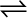7.17 Kp = 0.04 atm at 899 K for the equilibrium shown below. What is the equilibrium concentration of C2H6 when it is placed in a flask at 4.0 atm pressure and allowed to come to equilibrium?

C2H6 (g)C2H4 (g) + H2 (g)

NEET SOLUTION:

Let p be the pressure exerted by ethene and hydrogen gas (each) at equilibrium. Now, according to the reaction,

Hence, at equilibrium,